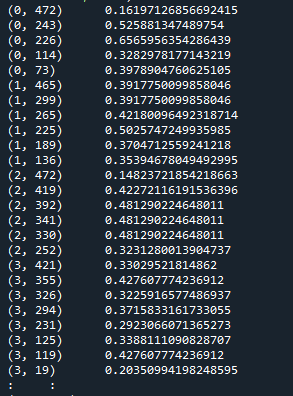# Sentimental Analysis using SVM

Sentimental analysis is the process of classifying various posts and comments of any social media into negative or positive. Using NLP(Natural Language Programming) or ML(Machine Learning) is the best way to make this process easier.

The project I did for sentimental analysis has the following program flow.

Steps to perform analysis-

The steps for any sentimental analysis is:-

1. Preparation of Data set– one can take any type of data or can download from net also. More the data more will be accuracy of the prediction.
2. Data pre processing– In this step we make the words simpler so that the prediction becomes easy. Some common data pre processing methods are- tokenization(dividing into each word),lemmitization,stemming and removing stop words(unwanted words) and characters.lemmitization means getting the original word of the input word that is “beautiful” will become “beauty”
3. Feature extraction-For all classification algorithms, features are necessary to either plot or make a precise detail so that the predictions are based on that features. here we will use TFIDF algorithm
4. Classifier algorithms– Here we use svm(support vector machine) but various others like naive bayes , regression,etc. can be used.
5. Prediction- Once all the above steps are done the model is ready to do the predictions. We will do the predictions on the testing dataset.

Necesary imports:-

The data set used is quite simple and is manually entered. The data set is a csv file. You can get a direct comments dataset on google. The data set is nearly of length 308.

Python Code –

Preparing Dataset-

```#the following line is used so that we run the program again and again the original input values are maintained.
np.random.seed(500)
#now lets read the data set using panda(pd)
#latin is used as the data set is long so to decode and proper start byte
data.dropna(inplace=True)#removing all empty spaces
# Change all the text to lower case.
#Python interprets ‘car’ and ‘CARS’ differently.I have not used stemming in this program but the process is simple and can be done by using in built functions like “ntlk”.
data[‘Sentence’] = [entry.lower() for entry in data[‘Sentence’]]
data[‘Sentiment’] = np.where(data[‘Sentiment’].str.contains(‘positive’), 1, 0)
#the above step divides the positive as 1 and negative as 0 this could have been done by label encoder but my train_y array is 1 d
Train_X, Test_X, Train_Y, Test_Y = train_test_split(data[‘Sentence’],data[‘Sentiment’],test_size=0.3)
#splitting the data set as training and testing sets in 70:30 ratio
print(Train_X.shape,Train_Y.shape)#this helps to view the number of rows in the data set

```

OUTPUT-

Classifying Sentiment in numeric form and performing TF-IDF

```Encoder = LabelEncoder()#this is used so that all the entries of Y is properly divided as 1 and 0
Train_Y = Encoder.fit_transform(Train_Y)
Test_Y = Encoder.fit_transform(Test_Y)
my_stopword=d.values.tolist() #converts the datatype to list
#removing the unwanted words like “are,is you,will,etc…”(stopwords.csv has the list of words)
#tfidf feature extraction using the function
vectorizer = TfidfVectorizer(my_stopword)
vectorizer.fit_transform(data[‘Sentence’])
#feature_names = vectorizer.get_feature_names() by this u can view if the stop words are removed and the only important feature words
#values of tfidf for train data and test data
Train_X_Tfidf = vectorizer.transform(Train_X)
Test_X_Tfidf = vectorizer.transform(Test_X)
print(Train_X_Tfidf)```(a,b) c :csr matrix : a- memory index b-unique binary value for each number c- tfidf value.

Perfroming SVM-

```#SVM function inbuilt in the library
SVM = svm.SVC(C=1.0, kernel=’linear’, degree=3, gamma=’auto’)
SVM.fit(Train_X_Tfidf,Train_Y)
# predict the labels on validation dataset
predictions_SVM = SVM.predict(Test_X_Tfidf)
# Use accuracy_score function to get the accuracy
print(“SVM Accuracy Score -> “,accuracy_score(predictions_SVM, Test_Y)*100)
#if you want to enter an input sentence and check the classificcation as positive or negative
lst = [ ]
print(“Enter sentences: “)

for i in range(0, 2):
ele = input()
lst.append(ele)

#print(lst)
tes=vectorizer.transform(lst)
#print(tes)
predictions= SVM.predict(tes)
#print(predictions)
for i in predictions:
if predictions[i] == 1 :
print(“ — — positive”)
else:
print(“ — — negative”)
```

OUTPUT-

Hope you all understood it!